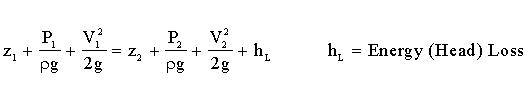Bernoulli Equation Calculator

Bernoulli Equation (Energy Equation) for Fluid Flow

 Solve for: Upstream Elevation Downstream Elevation Upstream Pressure Downstream Pressure Upstream Velocity Downstream Velocity Energy (Head) Loss Fluid Mass Density, ρ (slug/ft3): Select units: Upstream Elevation, Z1 (ft): British Gravitational (BG) units International System (SI) units Downstream Elevation, Z2 (ft): © 2014 LMNO Engineering, Upstream Pressure, P1 (lb/ft2): Research, and Software, Ltd. Downstream Pressure, P2 (lb/ft2): http://www.LMNOeng.com Upstream Velocity, V1 (ft/s): Downstream Velocity, V2 (ft/s): Energy (Head) Loss, hL (ft):

Units in Bernoulli calculator: ft=foot, kg=kilogram, lb=pound, m=meter, N=Newton, s=second.

Bernoulli (Energy) Equation for steady incompressible flow:Mass density ρ can be found at mass density of liquids and gases.
g = acceleration due to gravity = 32.174 ft/s2 = 9.806 m/s2.

The steady state incompressible energy equation (also known as the Bernoulli equation) models a fluid moving from location 1 to location 2. The loss term hL accounts for all minor (valves, elbows, etc.) and major (pipe friction) losses between 1 and 2. For Bernoulli applications, please see our Bernoulli Calculator with Applications.

The Bernoulli equation (energy equation) calculation does not check for unreasonable inputs such as negative density, negative velocity, or pressure less than a complete vacuum.

LMNO Engineering, Research, and Software, Ltd.
7860 Angel Ridge Rd.   Athens, Ohio 45701  USA   Phone: (740) 707-2614
LMNO@LMNOeng.com    http://www.LMNOeng.com

To:

Fluid Densities

Sophisticated related calculations:

Bernoulli Calculator with Applications

Pipe Design (Darcy-Weisbach friction losses)

Pipe Design (Hazen-Williams friction losses)

Simple related calculations:

Compute velocity from flow Q=VA

Energy loss using Darcy-Weisbach method

Energy loss using Hazen-Williams method

Unit Conversions

Other information:

Register

Discussion and References